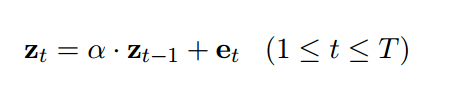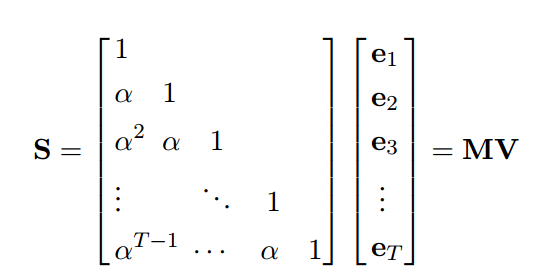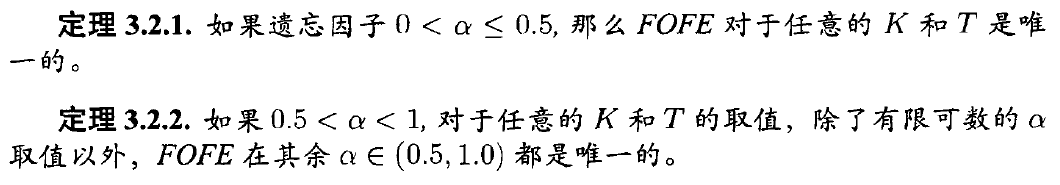|     |   0 浏览

## FOFE

FOFE(Fixed-size Ordinally Forgetting Encoding, 固定长度依次遗忘编码)是论文Zhang et. 2015中提出的一种将变长字符串进行定长表示的方法。

e_t为1_of_K编码，FOFE编码递归定义为：{ABC}关于C的编码为：[a^2 a 1]
{ABCBC}关于C的编码为：[a^4 a^3 + a a^2 + 1]

``````A = [1 0 0]
AB = a * [1 0 0] + [0 1 0]=[a 1 0]
ABC = a * [a 1 0] + [0 0 1] = [a^2 a 1]

ABCB = a * [a^2 a 1] + [0 1 0] = [a^3 a^2 + 1 a]
ABCBC = a * [a^3 a^2 + 1 a] + [0 0 1] = [a^4 a^3 + a a^2 + 1]
``````## 定理## 来源

• Zhang et. 2015
• 《基于深度神经网络的语音识别模型研究》